# JEE Main 2020 Physics Paper With Solutions Sept 6 (Shift 1)

JEE Main 2020 Paper with Solutions Physics - Shift 1 will help the students to prepare efficiently for the JEE Main exams by understanding the allocation of marks for different topics across the subjects. Practising JEE Main previous year question papers with answer keys will help the aspirants to face the exam confidently. This set of solutions is designed to help the candidates in achieving time management skills and also acts as an effective aid in their preparation.

### September 6 Shift 1 - Physics

Question 1: Four point masses, each of mass m, are fixed at the corners of a square of side l. The square is rotating with angular frequency 𝛚, about an axis passing through one of the corners of the square and parallel to its diagonal, as shown in the figure. The angular momentum of the square about this axis is: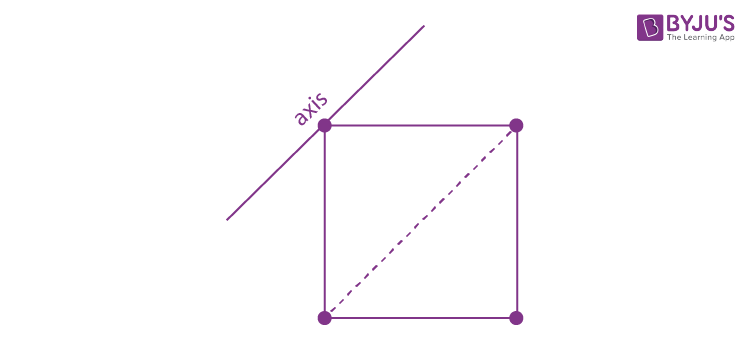1. 1) 4ml2ω
2. 2) 2ml2ω
3. 3) 3ml2ω
4. 4) ml2ω

Solution: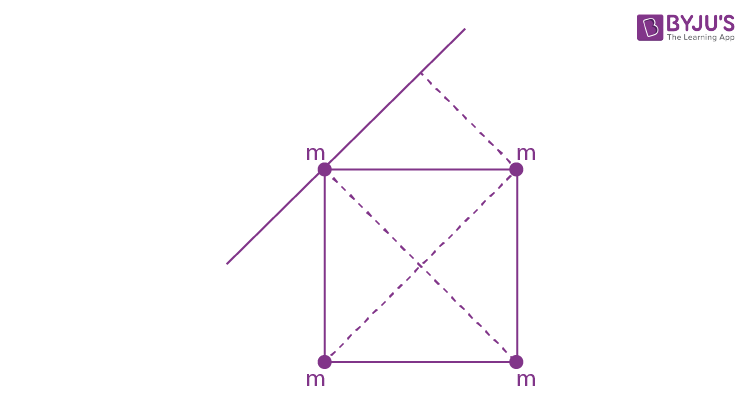L = Iω

I = m (a / √2)2 * 2 + m (√2a)2

= ma2 + 2ma2

∴ L = Iω = 3ml2ω (a = l)

Question 2: A screw gauge has 50 divisions on its circular scale. The circular scale is 4 units ahead of the pitch scale marking, prior to use. Upon one complete rotation of the circular scale, a displacement of 0.5mm is noticed on the pitch scale. The nature of zero error involved and the least count of the screw gauge, are respectively:

1. 1) Positive, 0.1 mm
2. 2) Positive, 0.1μ m
3. 3) Positive, 10 μm
4. 4) Negative, 2 μ m

Solution:

L.C = (0.5 / 50) mm = 1 * 10-5 m = 10μ m

Question 3: An electron, a doubly ionized helium ion (He++) and a proton are having the same kinetic energy. The relation between their respective de-Broglie wavelengths λe, λHe++ and λp is:

1. 1) λe > λp > λHe++
2. 2) λe > λHe++ > λp
3. 3) λe < λp < λHe++
4. 4) λe < λHe++ = λp

Solution:

λ = h / p = h / √(2mK . E)

mHe > mp > me

λHe < λp < λe

Question 4: For the given input voltage waveform Vin(t), the output voltage waveform Vo(t), across the capacitor is correctly depicted by: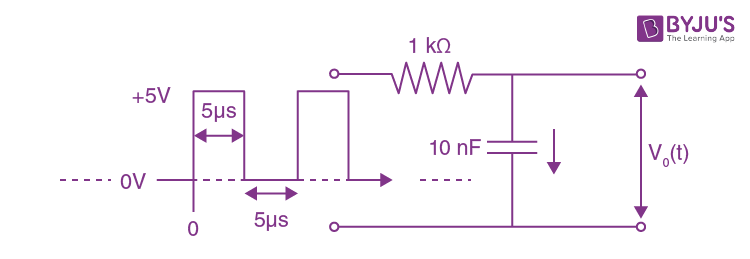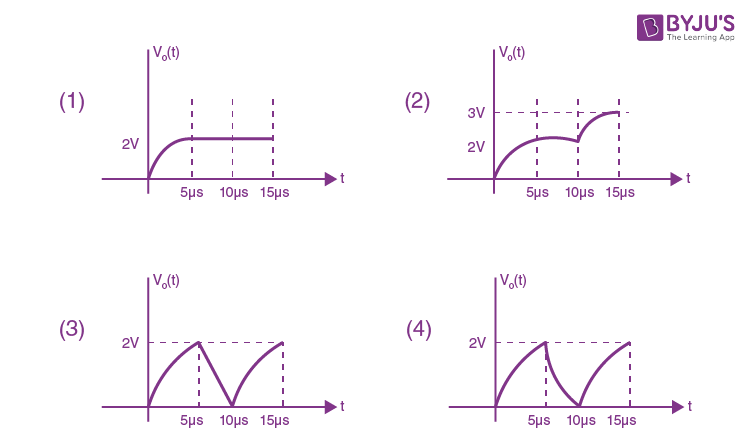Solution:

The answer is (2) because the capacitor is charging then discharging then again charging. But during discharging it is not possible to discharge 100%.

Question 5: Shown in the figure is a hollow ice cream cone (it is open at the top). If its mass is M, the radius of its top, R and height, H, then its moment of inertia about its axis is: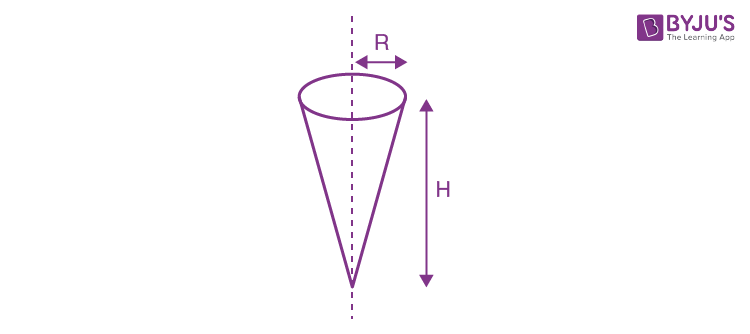1. 1) MR2 / 2
2. 2) MR2 / 3
3. 3) [M (R2 + H2) / 4]
4. 4) MH2 / 3

Solution:

I = MR2 / 2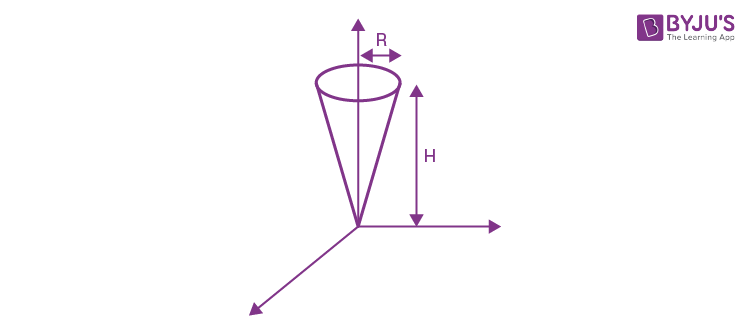Now cut a ring of thickness dx at distance y from the origin.

dI = dm r2

$$dm = \frac{M}{\pi Rl}(2\pi rdy)$$

Where dm is the mass of the elemental ring

$$I_{HC} = \int \frac{2M}{Rl} r^{3}dy$$

$$\frac{r}{R} = \frac{y}{l} \Rightarrow r = \frac{R}{l}y$$

$$I_{HC} = \frac{2M}{Rl} \frac{R^{3}}{l^{3}}\int_{o}^{l}y^{3}dy = \frac{MR^{2}}{2}$$

Question 6: A satellite is in an elliptical orbit around a planet P. It is observed that the velocity of the satellite when it is farthest from the planet is 6 times less than that when it is closest to the planet. The ratio of distances between the satellite and the planet at closest and farthest points is:

1. 1) 1 : 2
2. 2) 1 : 3
3. 3) 1 : 6
4. 4) 3 : 4

Solution:Li = Lf

m6vr1 = mvr2

6r1 = r2

⇒ (r1 / r2) = 1 / 6

Question 7: You are given that Mass of 73Li = 7.0160u,

Mass of 42He = 4.0026u and Mass of 11H = 1.0079u.

When 20 g of 73Li is converted into 42He by proton capture, the energy liberated, (in kWh), is: [Mass of nucleon = 1 GeV/c2]

1. 1) 6.82 × 105
2. 2) 4.5 × 105
3. 3) 8 × 106
4. 4) 1.33 × 106

Solution:

73Li + 1e+ → 2 42He

Δm ⇒ [mLi + mH] - 2 [MHe]

→ Δm = [7.0160 + 1.0079] - 2 × 4.0003

= 0.0187

Energy released in 1 reaction ⇒ Δmc2

In use of 7.016 u Li energy is ⇒ Δmc2

In use of 1gm Li energy is Δmc2 / mLi

In use of 20gm energy is ⇒ [Δmc2 / mLi] × 20gm

= [0.0187 × 931.5 × 106 × 1.6 × 10-19 × (20 / 7) × 6.023 × 1023] / [36 × 105]

= 1.33 × 106

Question 8: If the potential energy between two molecules is given by U = - (A / r6) + (B / r12), then at equilibrium, the separation between molecules, and the potential energy are:

1. 1) [2B / A], [-A2 / 4B]
2. 2) [2B / A], [-A2 / 2B]
3. 3) (B / A), 0
4. 4) (B / 2A), [-A2 / 4B]

Solution:

F = -dU / dr = (-d / dr) (-Ar-6 + Br-12) for equation F = 0

= [A (-6)] / r7 + [B . 12] / r13 = 0

[12B / r13] = [6A / r7]

r = (2B / A)

U = -A / [(2B / A)] + B / [(2B / A)2]

= [-A2 / (2B)] + [A2 / 4B]

= [-A2 / 4B]

Question 9: A clock has a continuously moving second’s hand of 0.1 m length. The average acceleration of the tip of the hand (in units of ms–2) is of the order of

1. 1) 10–3
2. 2) 10–1
3. 3) 10–2
4. 4) 10–4

Solution:

a = v2 / R v = 2πR / 60

= [4π2 . R2] / [602 . R] = [4π2R / 602] = (4 / 602) × 10 × 0.1 ≈ 10-3

Question 10: Identify the correct output signal Y in the given combination of gates (as shown) for the given inputs A and B.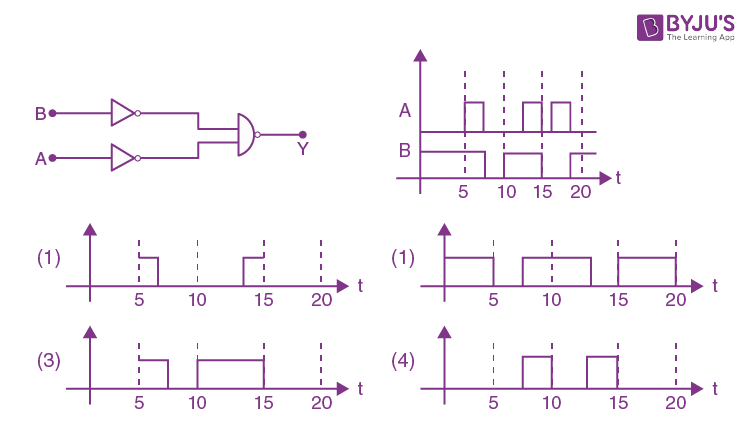Solution:

1. Answer: None of the options is correct.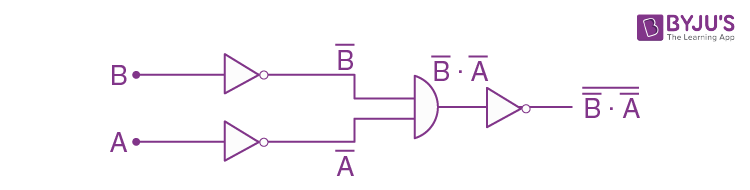Question 11: An electron is moving along +x direction with a velocity of 6 × 106 ms–1. It enters a region of the uniform electric field of 300 V/cm pointing along +y direction. The magnitude and direction of the magnetic field set up in this region such that the electron keeps moving along the x-direction will be

1. 1) 3 × 10–4 T, along –z direction
2. 2) 5 × 10–3 T, along –z direction
3. 3) 5 × 10–3 T, along +z direction
4. 4) 3 × 10–4 T, along +z direction

Solution:

B must be in the +z axis.qE = qVB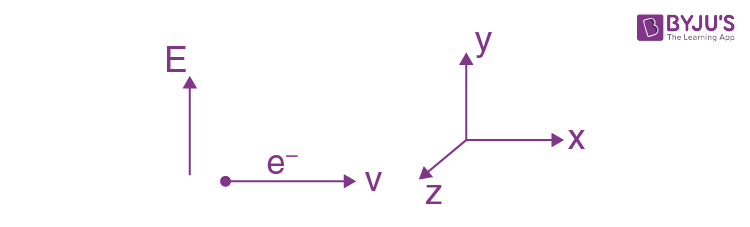E = 300 (v / 10-2m)

= 30000 v/m

B = E / V = [3 × 104] / [6 × 106] = 5 × 10-3 T

Question 12: In the figure below, P and Q are two equally intense coherent sources emitting radiation of wavelength 20 m. The separation between P and Q is 5 m and the phase of P is ahead of that of Q by 90°. A, B and C are three distinct points of observation, each equidistant from the midpoint of PQ. The intensities of radiation at A, B, C will be in the ratio: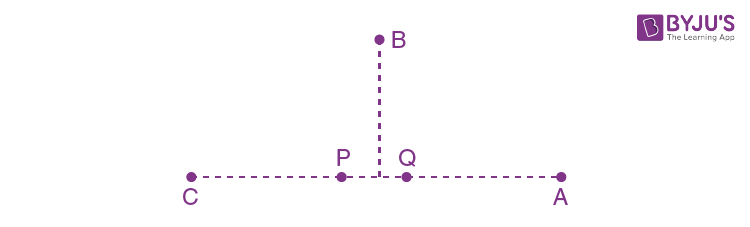1. 1) 4 : 1 : 0
2. 2) 2 : 1 : 0
3. 3) 0 : 1 : 2
4. 4) 0 : 1 : 4

Solution: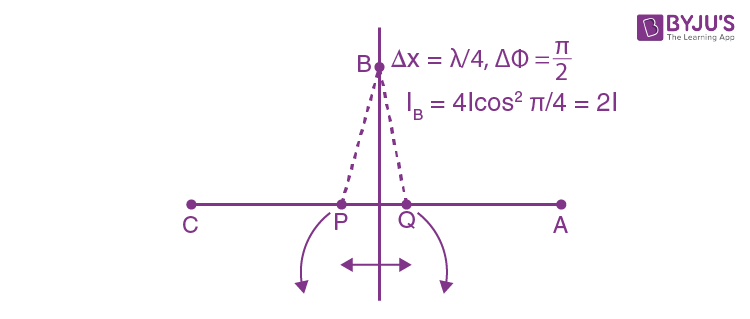Question 13: A point-like object is placed at a distance of 1 m in front of a convex lens of the focal length of 0.5 m. A plane mirror is placed at a distance of 2 m behind the lens. The position and nature of the final image formed by the system are:

1. 1) 1 m from the mirror, virtual
2. 2) 2.6 m from the mirror, virtual
3. 3) 1 m from the mirror, real
4. 4) 2.6 m from the mirror, real

Solution: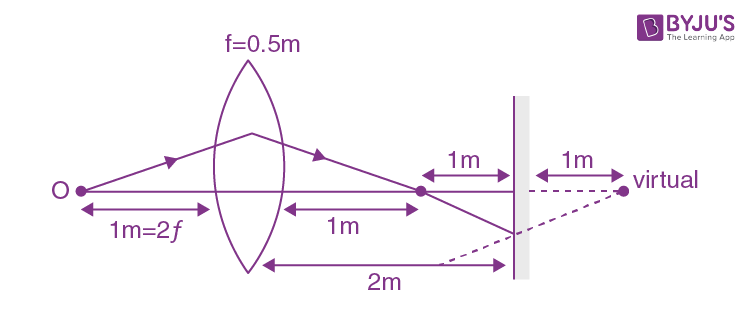For IIIrd Refraction, u = –3

(1 / v) + (1 / 3) = 2 / 1

V = 3 / 5 = 0.6

From mirror = 2.6m

Question 14: An insect is at the bottom of a hemispherical ditch of radius 1 m. It crawls up the ditch but starts slipping after it is at height h from the bottom. If the coefficient of friction between the ground and the insect is 0.75, then h is: (g = 10 ms–2)

1. 1) 0.45 m
2. 2) 0.60 m
3. 3) 0.20 m
4. 4) 0.80 m

Solution: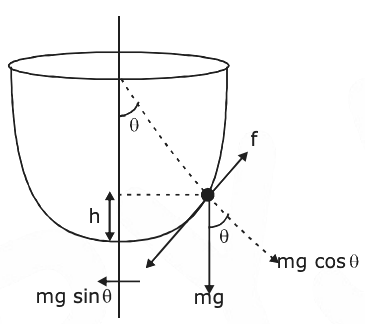f = mg sin θ

f = μmg cos θ

μmg cos θ = mg sin θ

tan θ = μ

tan θ = 3 / 4

cos θ = 4 / (√16 + 9) = 4 / 5

h = 1(1 cos θ) = 1 - (4 / 5) = 1 / 5

h = 1 / 5 = 0.2m

Question 15: Molecules of an ideal gas are known to have three translational degrees of freedom and two rotational degrees of freedom. The gas is maintained at a temperature of T. The total internal energy, U of a mole of this gas, and the value of γ [= Cp / Cv] are given, respectively by:

1. 1) U = [5 / 2] RT and γ = 7 / 5
2. 2) U = 5RT and γ = 6 / 5
3. 3) U = 5RT and γ = 7 / 5
4. 4) U = [5 / 2] RT and γ = 6 / 5

Solution:

U = [f / 2] nRT = (5 / 2) nRT =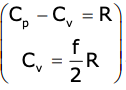, γ = [Cp / Cd] ⇒ 1 + (2 / f) = 1 + (2 / 5) = 7 / 5

Question 16: An object of mass m is suspended at the end of a massless wire of length L and area of cross-section A. Young modulus of the material of the wire is Y. If the mass is pulled down slightly its frequency of oscillation along the vertical direction is:

1. 1) f = (1 / 2π) √(YA / mL)
2. 2) f = (1 / 2π)) √(mL / YA)
3. 3) f = (1 / 2π)) √(YL / mA)
4. 4) f = (1 / 2π)) √(mA / YL)

Solution:

Y = [F / A] / [ΔL / L]

Y = FL / AΔL

F = YAΔL / L

f = (1 / 2π)) √(YA / mL) [yA / L = K] ∵T = 2π) √m / K

Question 17: An AC circuit has R = 100Ω, C = 2μF and L = 80 mH, connected in series. The quality factor of the circuit is:

1. 1) 20
2. 2) 2
3. 3) 0.5
4. 4) 400

Solution:

Q = ωL / R

ω = 1 / √LC

= L / R √LC = (1 / R) √L / C

= (1 / 100) √(80 × 10-3) / (2 × 10-6) = 2

Question 18: Charges Q1 and Q2 are at points A and B of a right angle triangle OAB (see figure). The resultant electric field at point O is perpendicular to the hypotenuse, then Q1 / Q2 is proportional to: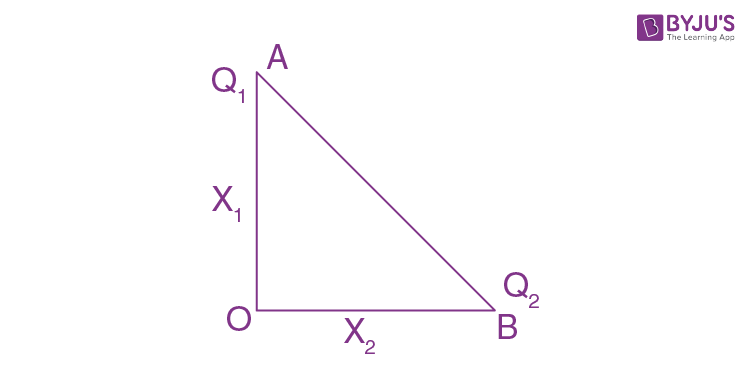1. 1) x2 / x1
2. 2) x22 / x12
3. 3) x31 / x23
4. 4) x1 / x2

Solution: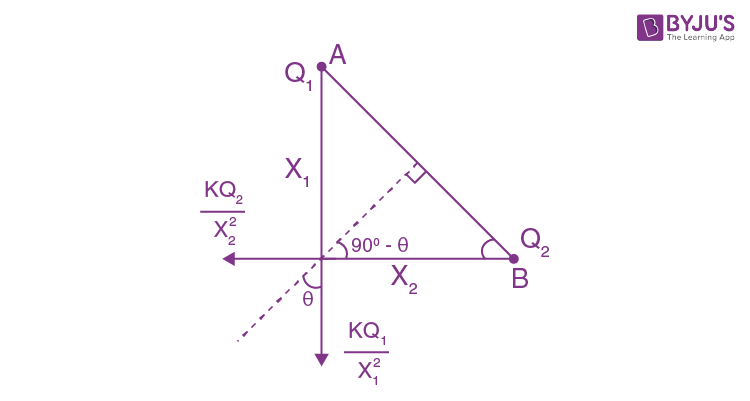tan θ = [kQ2 / x22] / [kQ1 / x12] = x1 / x2

[Q2 . x12] / [Q1 . x22] = X1 / X2

Q1 / Q2 = x1 / x2

Question 19: A sound source S is moving along a straight track with speed v, and is emitting, the sound of frequency vO (see figure). An observer is standing at a finite distance, at the point O, from the track. The time variation of frequency heard by the observer is best represented by (t0 represents the instant when the distance between the source and observer is minimum)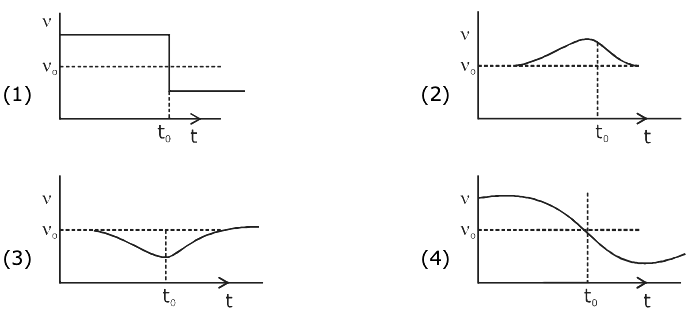Solution: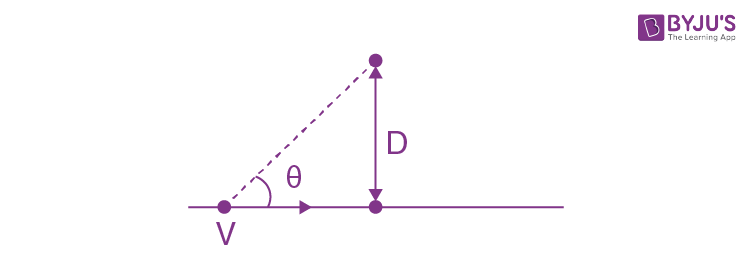fobserved ⇒ [Vsound / (Vsound - v cos θ)] f0

Initially, θ will be less ⇒ cos θ more

∴ fobserved more, then it will decrease.

Question 20: A particle of charge q and mass m is moving with velocity –vi (v ≠ 0) towards a large screen placed in the Y - Z plane at a distance d. If there is a magnetic field B = B0 k, the minimum value of v for which the particle will not hit the screen is:

1. 1) qdB0 / m
2. 2) qdB0 / 3m
3. 3) 2qdB0 / m
4. 4) qdB0 / 2m

Solution: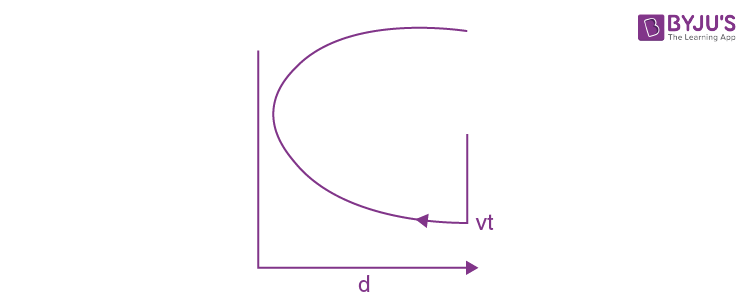d > R

d > (mv / qB0)

v < (qB0d / m)

Question 21: Two bodies of the same mass are moving with the same speed, but in different directions in a plane. They have a completely inelastic collision and move together thereafter with a final speed which is half of their initial speed. The angle between the initial velocities of the two bodies (in degree) is ________.

Solution: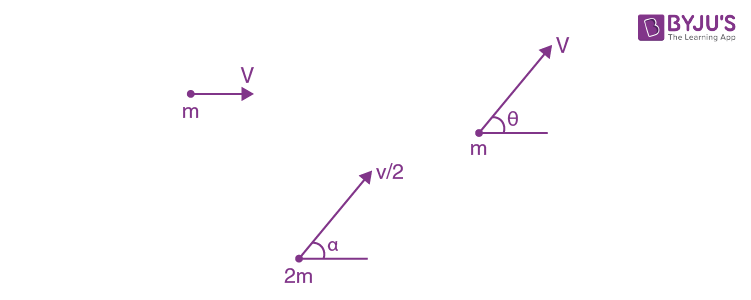∴ In the horizontal direction, by momentum conservation.

mv + mv cos θ = 2m (v / 2) cos ɑ

1 + cos θ = cos ɑ ------ (1)

In the vertical direction, by momentum conservation.

0 + mv sin θ = 2m (v / 2) sin ɑ

sin θ = sin ɑ

1 + cos θ = √1 - sin2 θ

θ = 120o

Question 22: Suppose that intensity of a laser is (315 / π) W/m2. The rms electric field, in units of V/m associated with this source is close to the nearest integer is __________. (∈ 0 = 8.86 × 10–12 C2Nm–2; c = 3 × 108 ms–1)

Solution:

$$I = \frac{1}{2}\varepsilon _{o}C E_{rms}^{2}$$

$$\frac{3.15}{\pi } = \frac{1}{2} \times 8.86\times 10^{-12\times3 \times10^{8}\times E_{rms}^{2}}$$

$$E_{rms}=275 V/m$$

Question 23: The density of a solid metal sphere is determined by measuring its mass and its diameter. The maximum error in the density of the sphere is [x / 100] %. If the relative errors in measuring the mass and the diameter are 6.0% and 1.5% respectively, the value of x is_______.

Solution:ρ = m / [(4 / 3)π (d / 2)3]

ρ = k . (m / d3)

log ρ = log K + log m - 3logd

Diff.

d ρ / ρ = dm / m - 3 (dd / d)

= 6.0 + 3 × 1.5 = 10.5%

= x = 1050

Question 24: Initially a gas of diatomic molecules are contained in a cylinder of volume V1 at a pressure P1 and temperature 250 K. Assuming that 25% of the molecules get dissociated causing a change in the number of moles. The pressure of the resulting gas at temperature 2000 K, when contained in a volume 2V1 is given by P2. The ratio P2 / P1 is ________.

Solution: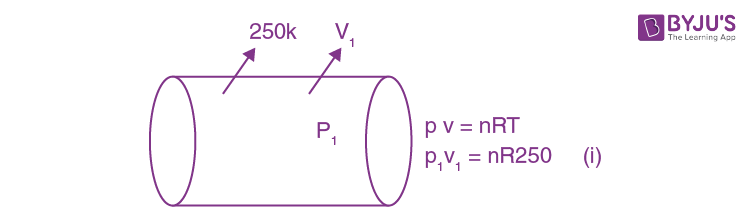25% will dissociate out of 100.

3n / 4 molecules will remain the same as S (n / 4) mole become → n / 2

∴ Total molecules used

→ (3n / 4) + (n / 2) = (5n / 4)

P22V1 = (5n / 4) . R . 2000 --- (ii)

Equation (ii / i)

2p2v1 / p1v1 = [5nR × 2000] / [4nR × 250]

(P2 / P1) = 5

Question 25: A part of a complete circuit is shown in the figure. At some instant, the value of current I is 1A and it is decreasing at a rate of 102 A s–1. The value of the potential difference VP – VQ, (in volts) at that instant, is _________.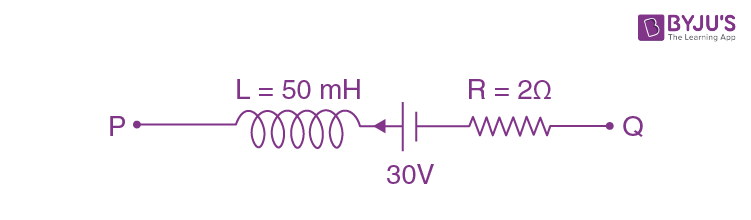Solution: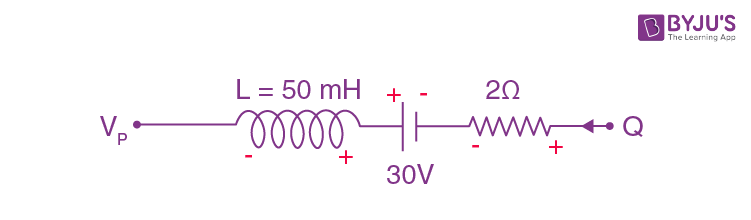VP + L . (di / dt) - 30 + 2i = VQ

VP + 50 × 10-3 (- 102) - 30 + 2 × 1 = vQ

VP – VQ = 35 - 2 = 33

### JEE Main 2020 Physics Paper With Solutions Shift 1 September 6